# Instructions

This handout shows and explains the code used in the lecture. You can use it to run the code on your own computer. Copy pasting the codes in the gray blocks and running it should produce the same outcomes.

# 1 Installing and activating packages

Don't forget to install package the first time using a package. You only have to install the package once.

install.packages("tidyverse")

Then activate the package each time you start a new session.

library("tidyverse")

Tip: you can use library() also to check whether a package is installed correctly. For instance run library("tidyverse") twice and if the second time no message is displayed then the package is correctly installed.

# 2 Graphics

## 2.1 Activate the graphics package ggplot2

ggplot2 is a graphics package and is part of the tidyverse. ggplot2 has been downloaded together with tidyverse and therefore we don't need to download is again.

library("ggplot2")

Open the data frame mpg. This is part of the ggplot2 package you just installed and activated.

mpg
# # A tibble: 234 x 11
#    manufacturer model      displ  year   cyl trans      drv     cty   hwy fl    class
#    <chr>        <chr>      <dbl> <int> <int> <chr>      <chr> <int> <int> <chr> <chr>
#  1 audi         a4          1.80  1999     4 auto(l5)   f        18    29 p     compact
#  2 audi         a4          1.80  1999     4 manual(m5) f        21    29 p     compact
#  3 audi         a4          2.00  2008     4 manual(m6) f        20    31 p     compact
#  4 audi         a4          2.00  2008     4 auto(av)   f        21    30 p     compact
#  5 audi         a4          2.80  1999     6 auto(l5)   f        16    26 p     compact
#  6 audi         a4          2.80  1999     6 manual(m5) f        18    26 p     compact
#  7 audi         a4          3.10  2008     6 auto(av)   f        18    27 p     compact
#  8 audi         a4 quattro  1.80  1999     4 manual(m5) 4        18    26 p     compact
#  9 audi         a4 quattro  1.80  1999     4 auto(l5)   4        16    25 p     compact
# 10 audi         a4 quattro  2.00  2008     4 manual(m6) 4        20    28 p     compact
# # ... with 224 more rows

mpg is a data set for the fuel economy data from 1999 and 2008 for 38 popular car models.

## 2.2 Histogram

With ggplot2 we can visualize the data. For instance we can assess the frequency of engine sizes with a histogram. The column displ is the engine displacement in liters, a common indicator for engine size.

ggplot(data = mpg) +
aes(displ) +
geom_histogram()
## stat_bin() using bins = 30. Pick better value with binwidth.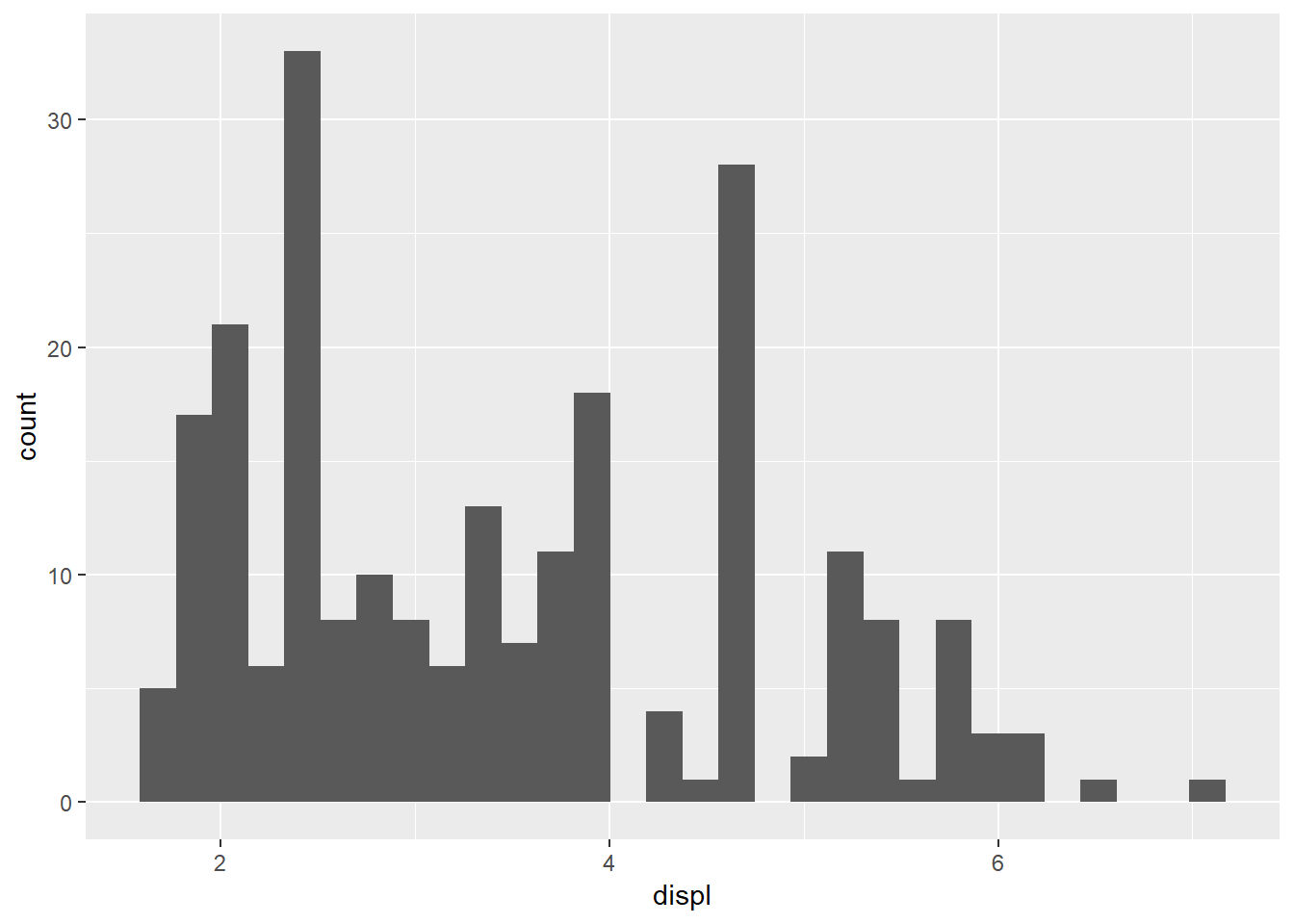## 2.3 Update labels and color

To make the histogram more readable we can change labels and colors.

ggplot(data = mpg) +
aes(x = displ) +
geom_histogram(fill = 'darkgreen') +
labs(title = "Histrogram of engine displacement",
x = "Engine displacement in litres",
y = "Frequency")
## stat_bin() using bins = 30. Pick better value with binwidth.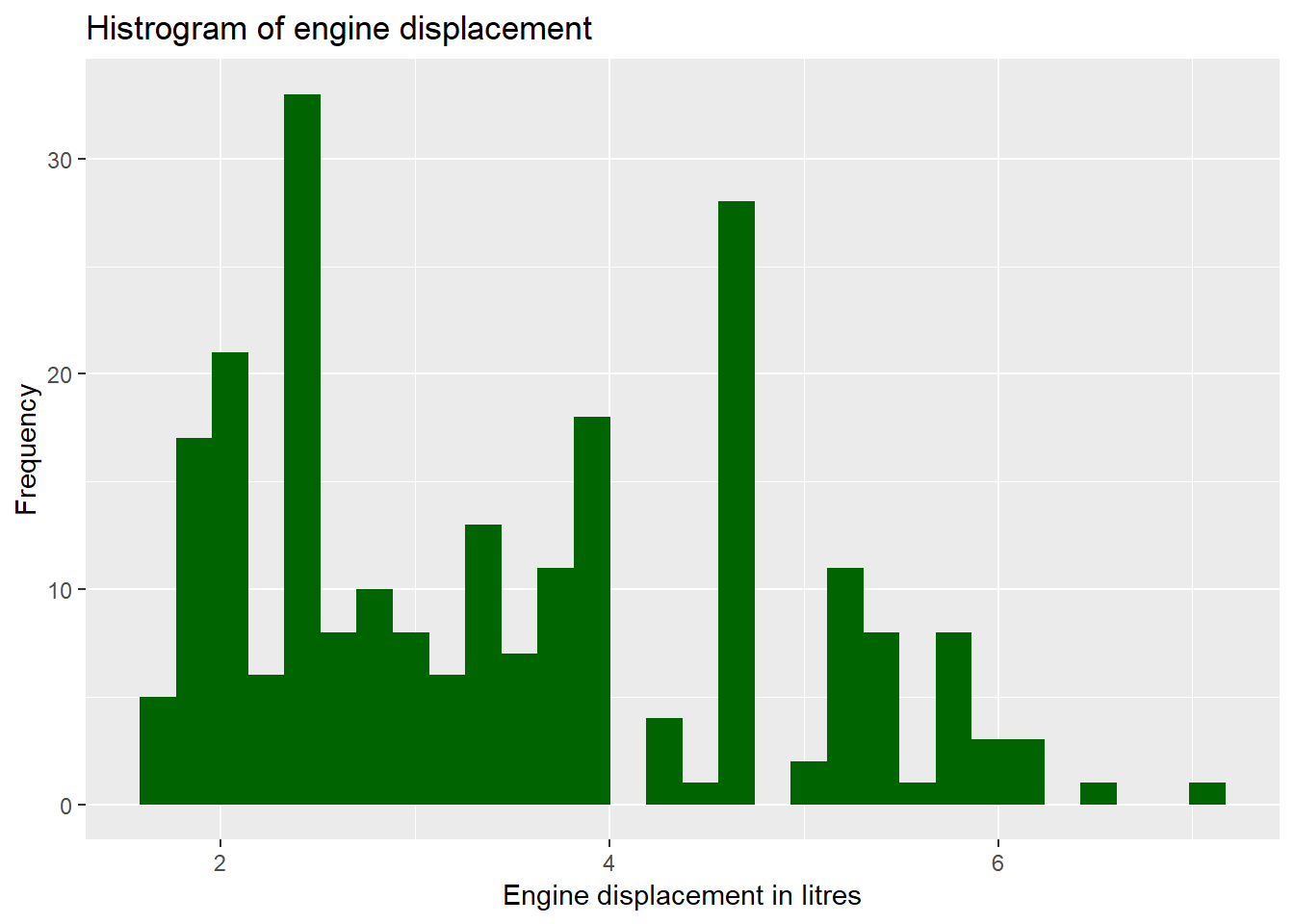## 2.4 Create a scatter dot

With a scatter dot we can get an idea if larger engines are more or less efficient on the highway. The column hwy are the burned miles per gallon on a highway.

ggplot(data = mpg) +
aes(x = displ, y = hwy) +
geom_point()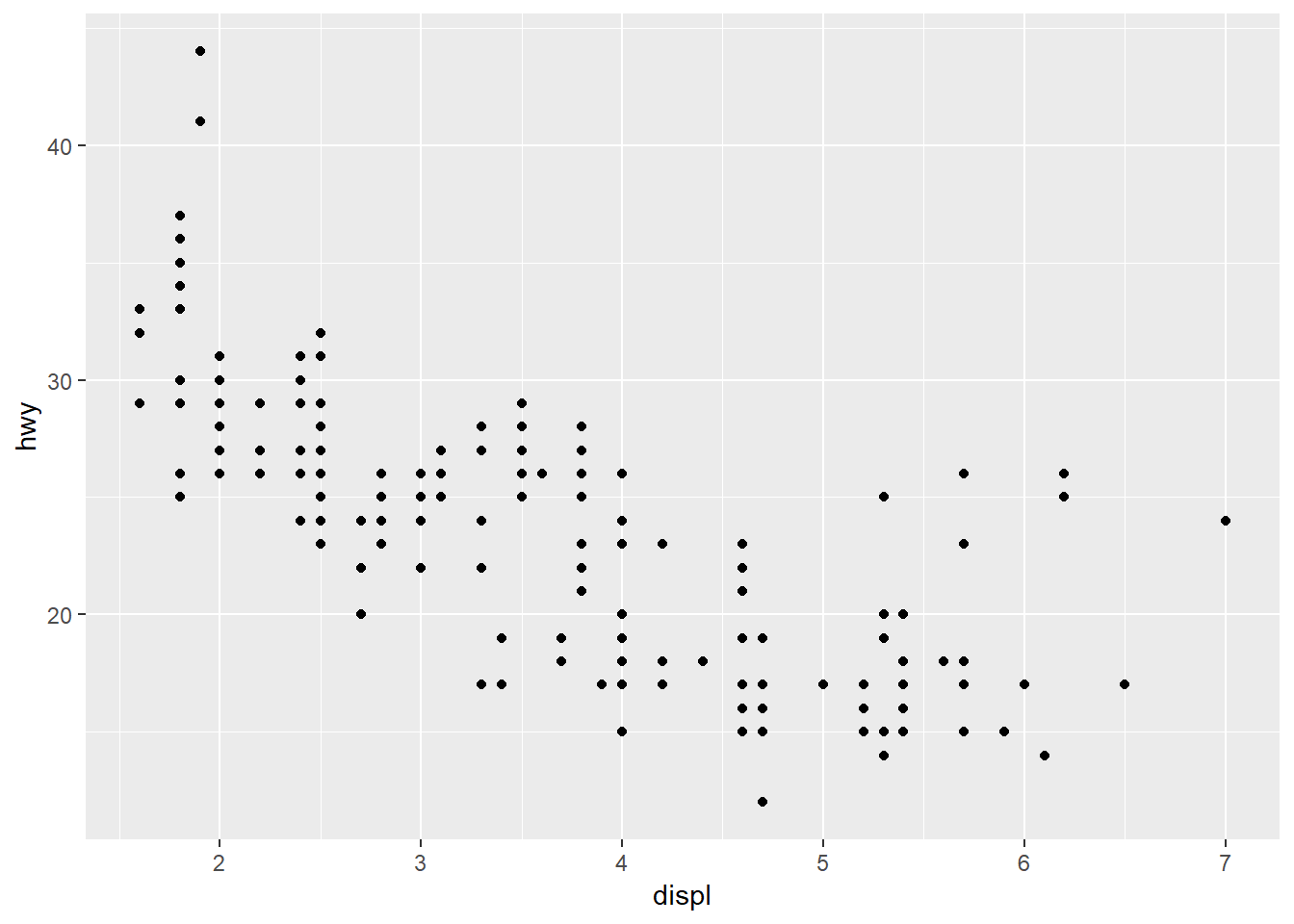## 2.5 Adding more aesthetic mappings

To get an idea how different car classes are performing we can give them different colors.

ggplot(data = mpg) +
aes(x = displ, y = hwy, color = class) +
geom_point()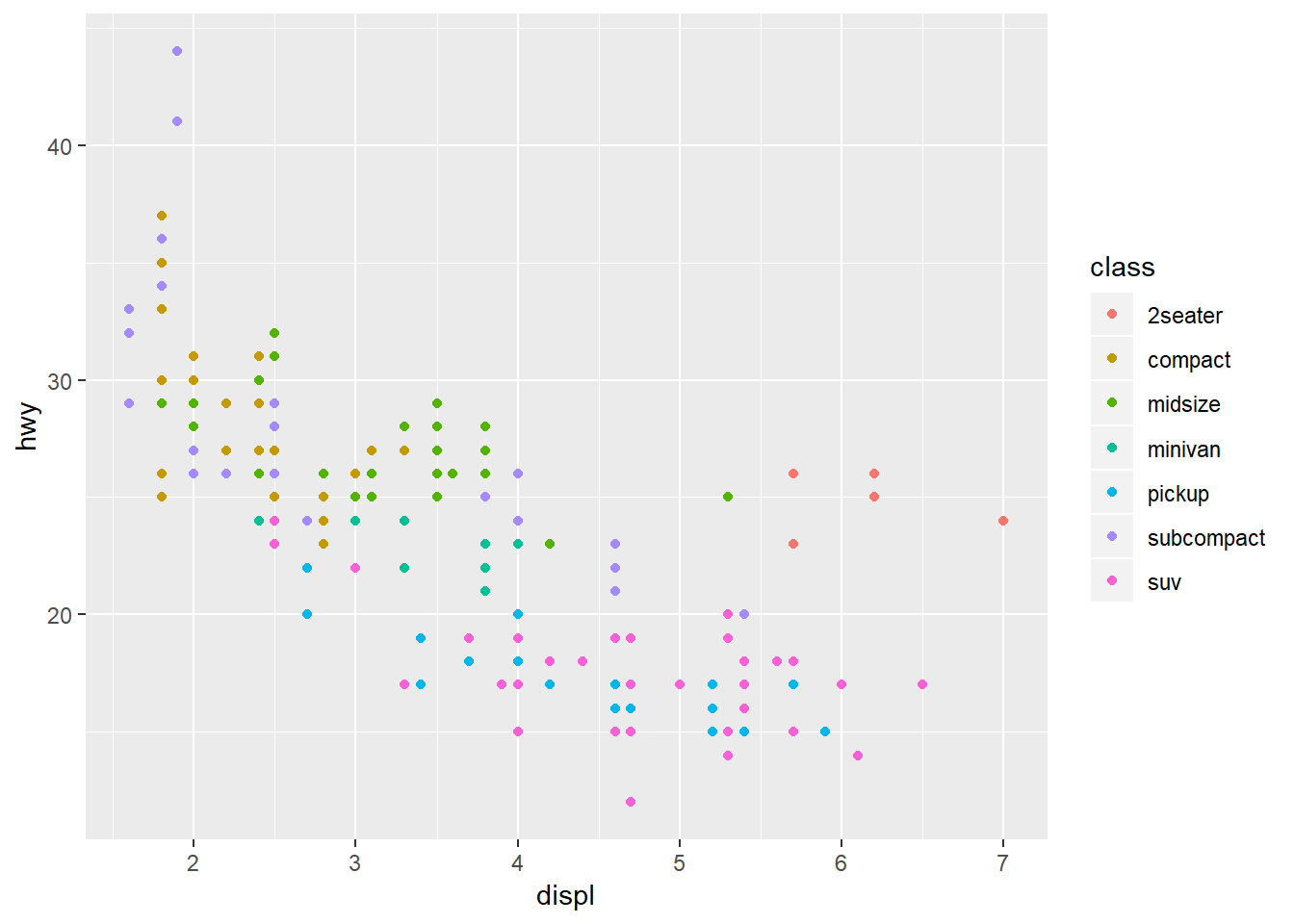In statistics we are often interested into linear analysis. For that we can also add a regression line to the scatter dot.

ggplot(data = mpg) +
aes(x = displ, y = hwy) +
geom_point() +
geom_smooth(method=lm)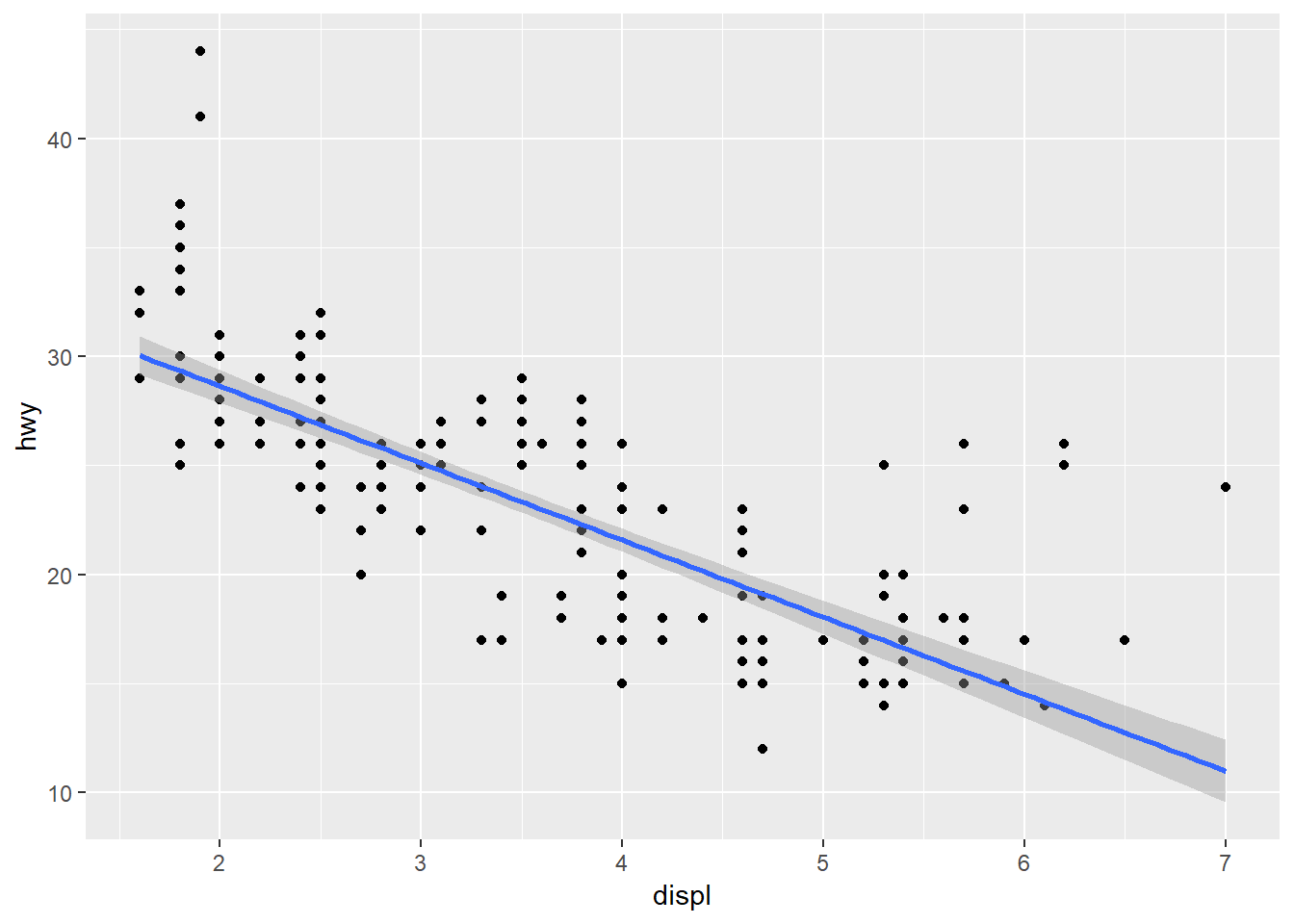What does this graph tell us?

# 3 Statistics

## 3.1 Descriptive statistics

You can get descriptive statistics with summary().

summary(mpg)
##  manufacturer          model               displ            year
##  Length:234         Length:234         Min.   :1.600   Min.   :1999
##  Class :character   Class :character   1st Qu.:2.400   1st Qu.:1999
##  Mode  :character   Mode  :character   Median :3.300   Median :2004
##                                        Mean   :3.472   Mean   :2004
##                                        3rd Qu.:4.600   3rd Qu.:2008
##                                        Max.   :7.000   Max.   :2008
##       cyl           trans               drv                 cty
##  Min.   :4.000   Length:234         Length:234         Min.   : 9.00
##  1st Qu.:4.000   Class :character   Class :character   1st Qu.:14.00
##  Median :6.000   Mode  :character   Mode  :character   Median :17.00
##  Mean   :5.889                                         Mean   :16.86
##  3rd Qu.:8.000                                         3rd Qu.:19.00
##  Max.   :8.000                                         Max.   :35.00
##       hwy             fl               class
##  Min.   :12.00   Length:234         Length:234
##  1st Qu.:18.00   Class :character   Class :character
##  Median :24.00   Mode  :character   Mode  :character
##  Mean   :23.44
##  3rd Qu.:27.00
##  Max.   :44.00

## 3.2 Correlation

For a better correlation analysis we need the package Hmisc.

install.packages("Hmisc")
library("Hmisc")

We can correlate engine size with highway fuel consumption with rcorr for the Hmisc package.

rcorr(x = mpg$displ, y = mpg$hwy)
##       x     y
## x  1.00 -0.77
## y -0.77  1.00
##
## n= 234
##
##
## P
##   x  y
## x     0
## y  0

## 3.3 Independent T-Test

To run a T test you can use t.test(x, y).

t.test(x = mpg$displ, y = mpg$hwy)
##
##  Welch Two Sample t-test
##
## data:  mpg$displ and mpg$hwy
## t = -50.131, df = 254.89, p-value < 2.2e-16
## alternative hypothesis: true difference in means is not equal to 0
## 95 percent confidence interval:
##  -20.75280 -19.18395
## sample estimates:
## mean of x mean of y
##  3.471795 23.440171

Tip: T test, anova an regression are all general linear models. Therefore you could also use t.test(y ~ x) where x is a factor with two levels giving the corresponding groups.

## 3.4 One Way Anova

The code for an ANOVA is fit <- aov(y ~ x, data = mydata) For our example that would be ...

fit <- aov(hwy ~ displ, data = mpg)
summary(fit)
##              Df Sum Sq Mean Sq F value Pr(>F)
## displ         1   4848    4848   329.5 <2e-16 ***
## Residuals   232   3414      15
## ---
## Signif. codes:  0 '***' 0.001 '**' 0.01 '*' 0.05 '.' 0.1 ' ' 1

## 3.5 Multiple Linear regression

The code for a regression is fit <- lm(y ~ x1 + x2 + x3, data = mydata). For our example that would be ...

fit <- lm(hwy ~ displ, data = mpg)
summary(fit)
##
## Call:
## lm(formula = hwy ~ displ, data = mpg)
##
## Residuals:
##     Min      1Q  Median      3Q     Max
## -7.1039 -2.1646 -0.2242  2.0589 15.0105
##
## Coefficients:
##             Estimate Std. Error t value Pr(>|t|)
## (Intercept)  35.6977     0.7204   49.55   <2e-16 ***
## displ        -3.5306     0.1945  -18.15   <2e-16 ***
## ---
## Signif. codes:  0 '***' 0.001 '**' 0.01 '*' 0.05 '.' 0.1 ' ' 1
##
## Residual standard error: 3.836 on 232 degrees of freedom
## Multiple R-squared:  0.5868, Adjusted R-squared:  0.585
## F-statistic: 329.5 on 1 and 232 DF,  p-value: < 2.2e-16